# DMC Busbar Insulation Spacer for Busduct Copper Duct Joint•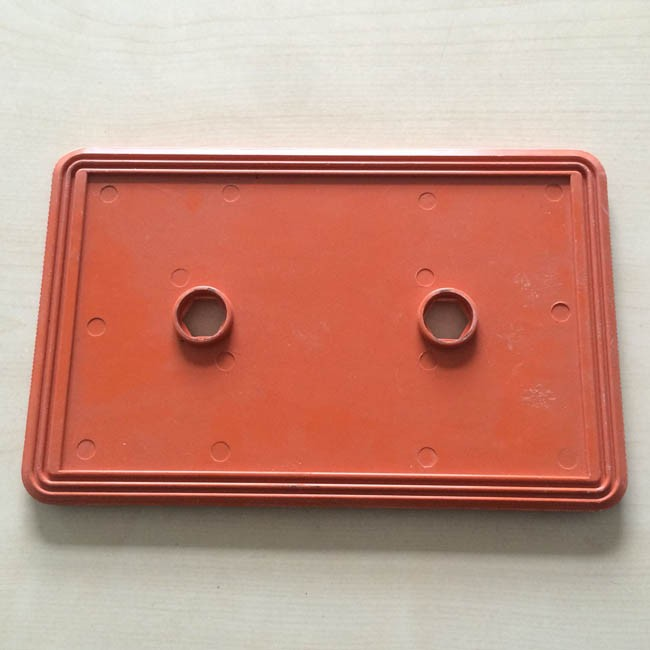•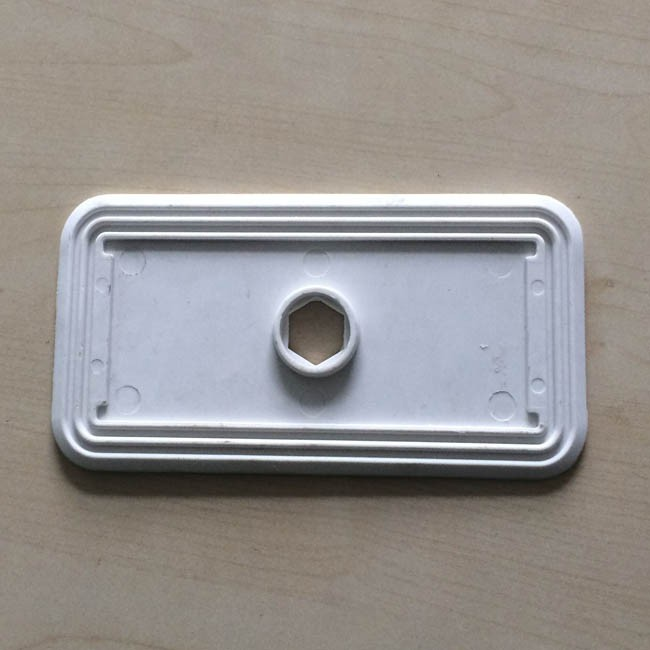•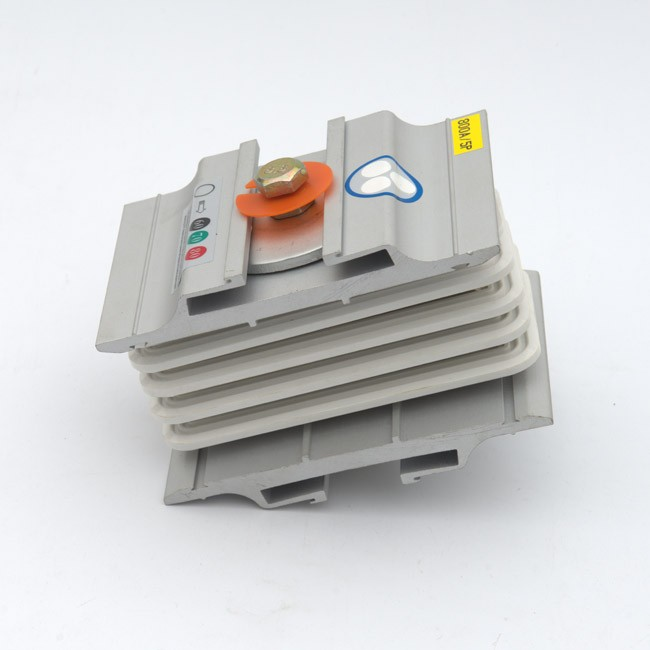DMC Busbar Insulation Spacer for Busduct Copper Duct Joint
• Kiande
• China
• 7-15 Days
• 1000 Per Month

DMC Busbar Insulation Spacer,Busbar Insulation Spacer, Duct Joint Insulation Spacer,
Insulation Spacer for Duct Joint,Busduct Insulation Spacer
With good insulation

DMC Busbar Insulation Spacer for Busduct Copper Duct Joint

Busbar joint includes double head bolt with but, insulation separator, copper bar, die casting end and bowl washer etc.

The busbar joint is used to connect two busbar trunking systems for current transmission.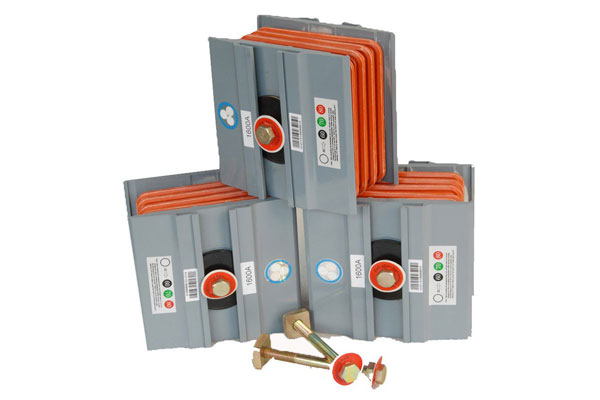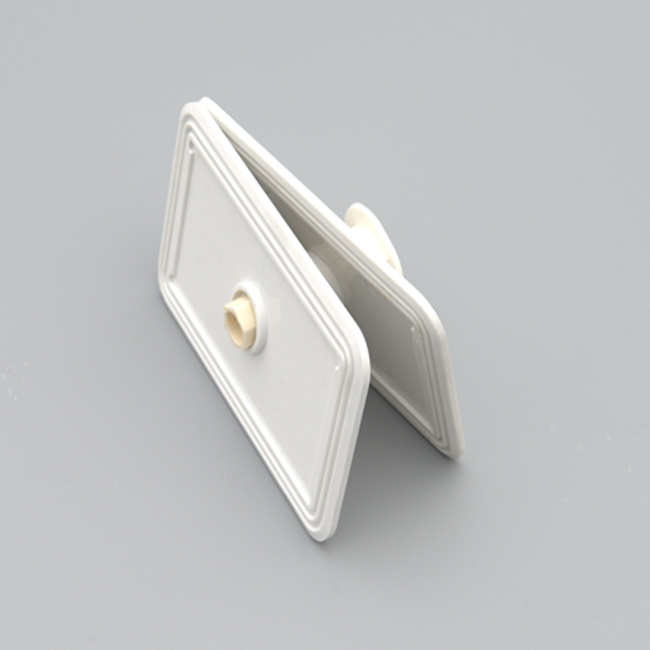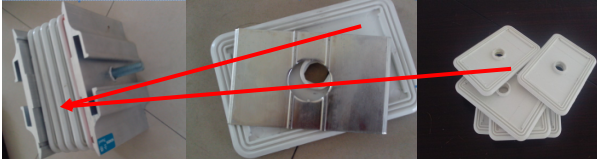Product Model Hole Nos Distance Between Holes X=122 Insulation Plate(Middle, Edge)X=122 X=50 1 X=65 1 X=85 1 X=90 1 X=105 1 X=120 1 X=140 1 X=150 2 80(RU) X=160 1 X=190 1 X=205 3 80(RU) X=210 2 105 X=230 2 115 X=250 2 125 X=255 3 85 X=310 2 169 X=350 2 189 X=390 2 209 X=450 4 95 X=490 4 B=105  C=154(Middle) X=658 6 B=90(Middle) C=139 (Edge) X=688 6 B=100(Middle) C=150(Edge) Insulation Plate(Middle, Edge)X=130 X=50 1 X=60 1 X=80 1 X=95 1 X=110 1 X=120 1 X=125 1 X=145 1 X=160 1 X=180 2 90 X=190 2 85 X=200 2 100 X=324 2 194 X=344 2 204 X=354 2 209 X=394 2 229 X=424 2 244 X=464 4 B=174 C=90 X=484 4 B=179 C=95 X=504 4 B=184 C=100 X=623 6 B=149 C=80 X=648 6 B=164 C=80

Suzhou Kiande Electric Co.,Ltd. is a comprehensive scientific enterprise specialized in intelligent production and data analysis for electricity manufacturing field. Company’s head office is located in Suzhou(Paradise on earth) and manufacturing center is in Zhen Jiang(Chinese electricity island).It covers R&D dept, design dept., production dept., sales dept. and after-service dept. which improves to provide better programs for production efficiency, quality, cost and data.

What is Busduct Joint component?

Joint block is operatable by one / two shear off bolts.

Joint assembly can be removed / installed at any time in installed condition.

Disc spring washer are used in Uniblock joint to uniformly distribute pressure.

Recommended torque for tightening is 7 Kg-mtr.

These joints are specially designed to accommodate thermal expansion of busbars and housing.

Product Tag:
Get the latest price? We'll respond as soon as possible(within 12 hours)